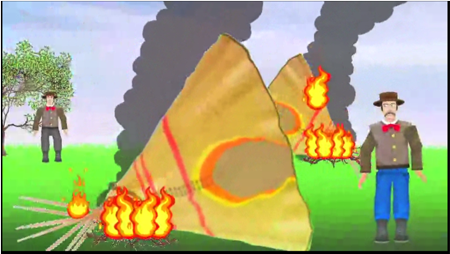# Watch me work: Finishing the test scoring with more SAS character functions

Recall that in the last post we were using SAS functions to score a test that had been completed by middle school and upper elementary students. Since we wanted to make it as easy as possible for students to enter their answers, we accepted just about any format.

Picking up where we left off …

In one question, the correct answer is 1/8 . Students entered 1/8, 1/8 cup, 1/8 cup of beans, and so on.  To score these, we use the substr function to read the first 3 characters and score the problem correct if those are “1/8”

If substr(q22,1,3) = “1/8” then q22 = 1 ;

else q22 = 0 ;

MISSING FUNCTION – TO CHECK FOR MISSING DATA

For q27, students clicked on which of the equations are correct. If they clicked the correct equation, the variable was set  to 1.

When they didn’t click on anything, it was missing. I wanted that to be changed to a zero, so I used the missing function, like this:

if missing(q27) then q27 = 0 ;

ARRAY, DO-LOOP and INPUT FUNCTION

Now, SAS is not the newest kid on the block

and I can relate, because neither am I, not even if I’m on a relatively old blockThe problem with being an older language is that  you have static types and you cannot have mixed arrays. What does that mean? It means that if you have defined q1 as a character variable because it might have a \$ in it then by God it is going to stay a character variable and you can’t be doing any funny stuff like finding the mean and standard deviation of it. Also, if you are going to have an array, everything in it better be either all character variables or all numeric variables.

Well, fine, then, here is how I change all those now scored questions to numeric. First, I created a new numeric array of 32 items. You can tell it is numeric because there is no \$.

Second, I used a DO loop and the INPUT function. The input function will read in a variable and and read it out in a different format, in this case, a numeric variable with a length of 8 and 0 decimal places.

I dropped the index variables j and i , which I mentioned in a previous post.

Now that I have my variables all nicely numeric, I can use the SUM function and add up all of the scored items into a total score.

array items {32} item1 – item32 ;

do j = 1 to 32 ;
items{j} = input(qs{j},8.0);
end;
drop i j ;
total = sum(of item1-item32);

Now that I have my data, the fun stuff begins, but that’s for another post because I need to get back to making games.

This is my day job. Check it out. Buy a game. Maturity is over-rated!Or donate a game to a school for good karma.